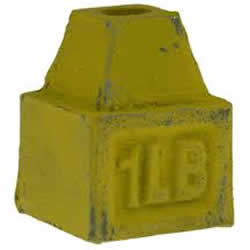# Difference between Kilos and Pounds

##### Key Difference: Kilo (Kilogram) is purely a unit of mass measurement. Whereas, pound can either be a unit of weight or force measurement.Differentiating between Kilos (kilograms) and Pounds is not such a difficult task. But, in the technical term it somehow becomes difficult for one to differentiate between both the units.

A kilogram (kg) is 2.2 times heavier than a pound (lbs), which means that one kilo of mass is equal to 2.2 lbs. One kilogram is the standard unit of measurement of weight. A kilogram weighs 9.81 Newtons (N) or 2.21 pounds (lb). The kilogram is not the weight of a person, and at the same time it is to be measured as a unit of mass.Pound is a unit of measurement that is popular in the imperial system, used most commonly by the United States. In order to differentiate it from the currency of Britain, also known as Pound, the unit of measurement is often abbreviated as ‘lb’ or ‘lbs’. According to the international convention there is only ‘lb’ and it does not have a plural form (i.e. ‘lbs’), though it has been gaining popularity in usage.

Comparison between Kilos and Pounds:

 Kilos Pounds Description (Dictionary.com) A unit of mass equal to 1000 grams: the base SI unit of mass, equal to the mass of the international prototype of the kilogram, a platinum-iridium cylinder kept in Sèvres, France. A unit of force and weight, equal to the force that produces an acceleration of 9.80665 meters per second per second when acting on a mass of one kilogram. A unit of weight and of mass, varying in different periods and countries. Abbreviation kg lb, lbm Unit Metric unit Imperial unit Derived Greek word Germanic word, libra pondo Meaning Thousand A pound by weight Equals to One kilogram is equal to 2.2046 pounds. International Avoirdupois Pound is equal to 0.4535 kilograms Unit of Kilogram is purely a unit of mass measurement. Pound can either be a unit of weight measurement

Image Courtesy: theguardian.com, jehecomfort.files.wordpress.com